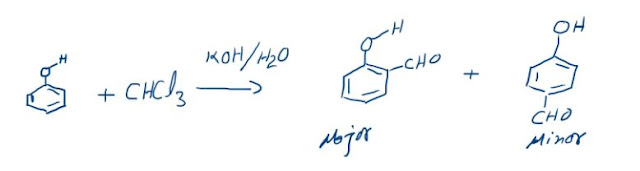## Plancks Quantum Theory

According to Planck’s Quantum Theory, energy is emitted in form of radiations from a source in a discontinuous manner that is in form of packets of energy. And energy of each packet depends on the frequency of radiation. These packets are called “Quantum of energy”. And at a time, only one quantum can be supplied to one electron or any other particle. And one Quantum cannot be divided or distributed. For example, if we take light, then we can consider it to be group of chocolates. Two rules guide the transfer of chocolates to students in a class. At a time a student gets only one chocolate, and a chocolate cannot be divided into two parts. These packets of energy are just like these chocolates. An electron can absorb one packet at a time and get excited. And the energy of a Quantum increases with frequency.
Energy of Quantum α Frequency
E α ν
The constant of proportionality is called Planck’s constant represented by “h”. So the equation becomes:
E = h ν
Where E is in J and the units of h are J s (Joule-seconds). The value of h = 6.626 × 10-34 Js. This expression gives the energy of one quantum. The radiation is a group of Quanta, that is, if it contains n Quanta of the same frequency then the total energy will become:
E = n hν
This is also called “Quantisation of Energy”.
This can also be expressed in terms of wavelength:
E = n hc/λ
We can also conclude that energy transferred by an electromagnetic radiation is an integral multiple of hν. That is, at an instant energy transferred to an electron cannot be 0.5hn and more than one packet cannot be absorbed by an electron. If more packets are incident then more electrons will be attacked by every packet of energy.

Note: Quantum of light is called “Photon”.

We can also conclude that energy transferred by an electromagnetic radiation is an integral multiple of hν.

### The Reimer-Tiemann reaction: Reaction and its Mechanism

Reimer-Tiemann Reaction Phenols on reaction with chloroform in the presence of sodium hydroxide (or potassium hydroxide) solution give hydro...